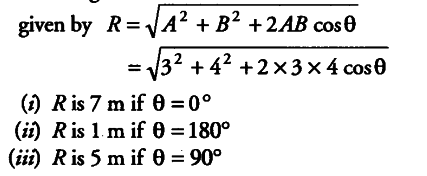# There are two displacement vectors

There are two displacement vectors, one of magnitude 3 m and the other of A m. How would the two vectors be added so that the magnitude of the resultant vector be (i) 7 m (ii) lm and (iii) 5 m

The magnitude of resultant R of two vectors A and B is given by